Function Repository Resource:

# SphericalLinearInterpolation

Evaluate the piecewise spherical linear interpolant of given data

Contributed by: Jan Mangaldan
 ResourceFunction["SphericalLinearInterpolation"][data,t] finds a piecewise spherical linear interpolation of data at the point t. ResourceFunction["SphericalLinearInterpolation"][data] represents an operator form of ResourceFunction["SphericalLinearInterpolation"] that can be applied to an expression.

## Details

Spherical linear interpolation is also known as "slerp".
ResourceFunction["SphericalLinearInterpolation"][data,t] evaluates so as to agree with data at every point explicitly specified in data.
data should be in the form {{t1,v1},{t2,v2},}, where the ti must be real numbers, and the vi must be vectors on a sphere, all of the same dimension.

## Examples

### Basic Examples (3)

Generate some data over a sphere of radius 3:

 In:=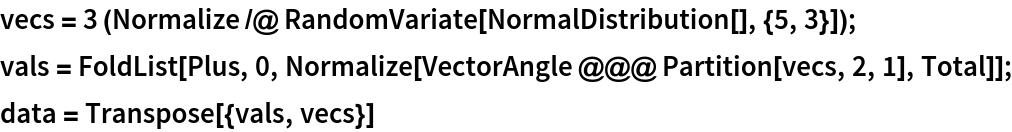Out=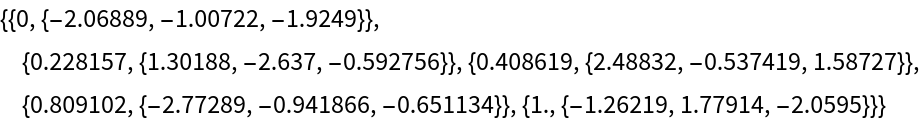Evaluate the piecewise spherical linear interpolant of the data at a given value:

 In:=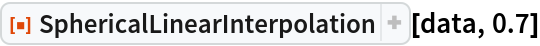Out=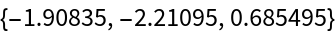Plot the piecewise spherical linear interpolant along with the original data:

 In:=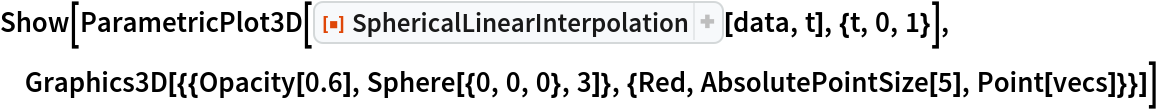Out=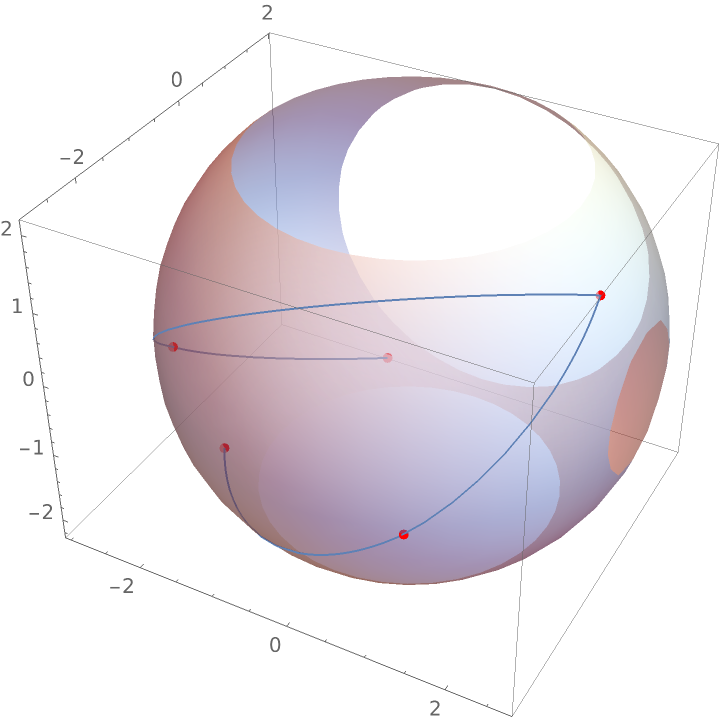### Scope (2)

Evaluate the piecewise spherical linear interpolant for four-dimensional vectors:

 In:=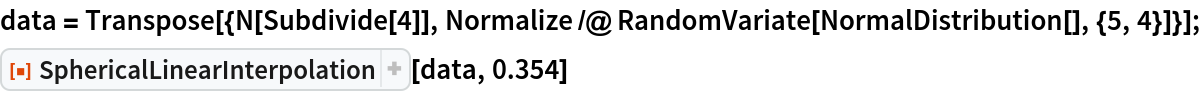Out=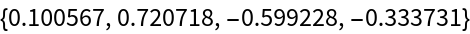Evaluate the piecewise spherical linear interpolant for high-precision data:

 In:=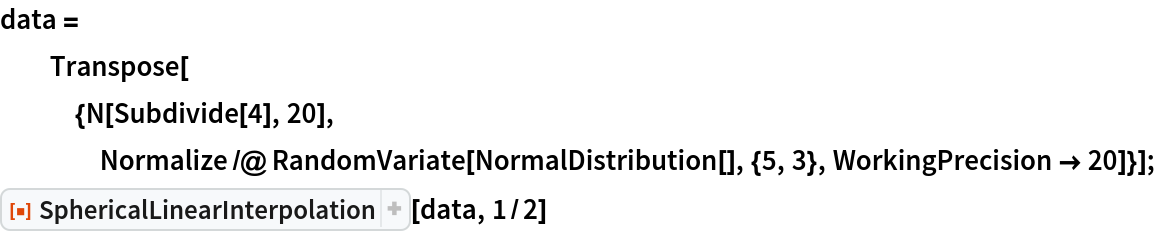Out=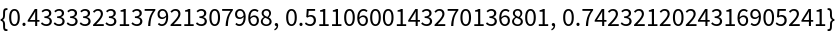### Applications (2)

A function for taking a bounded random step on a sphere:

 In:=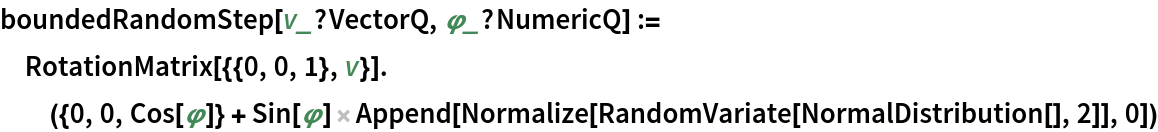Visualize a random walk with bounded steps on a sphere:

 In:=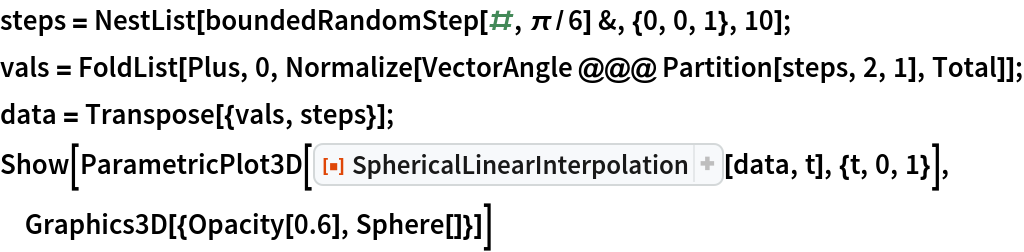Out=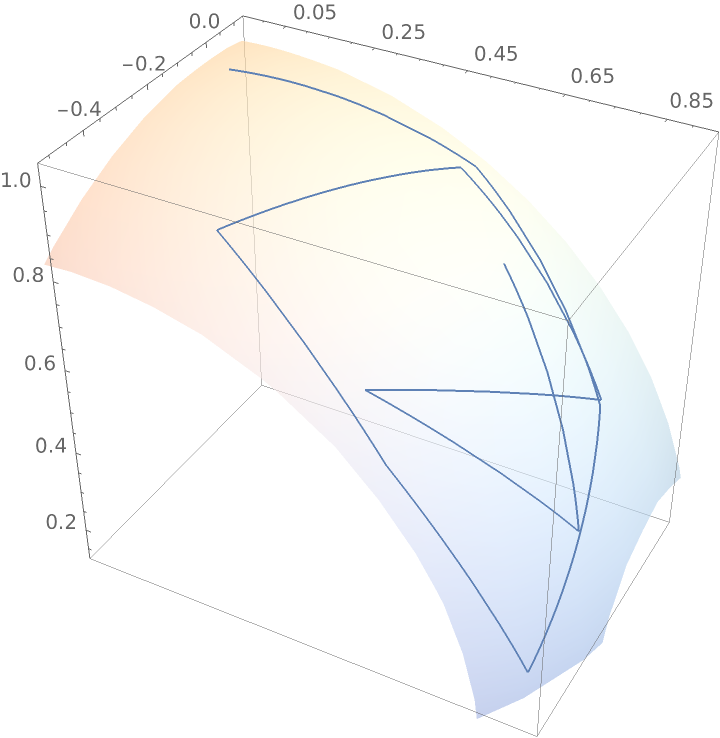## Version History

• 1.0.0 – 11 January 2021